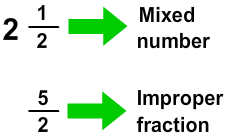# Convert Mixed Numbers to Improper Fractions

Print Rate 0 stars Common Core
Lesson size:
Message preview:
Someone you know has shared lesson with you:

To play this lesson, click on the link below:

https://www.turtlediary.com/lesson/convert-mixed-numbers-to-improper-fractions.html

Hope you have a good experience with this site and recommend to your friends too.

Login to rate activities and track progress.
Login to rate activities and track progress.

## Mixed Numbers

A mixed number is a combination of a whole number and a fraction, for example: 2
1
2
.

## Improper Fractions

A fraction in which the numerator is larger than or equal to the denominator, like
5
2
,
17
3
, or
6
6
is called an improper fraction.

## Convert Mixed Numbers to Improper Fractions

A mixed number can be expressed as a fraction.• Multiply the whole number by the denominator.
• Then add the result to the numerator.
• This result becomes new numerator.
• The denominator remains the same.

Let's illustrate through examples.

### Example 1

Write 2
1
2
as an improper fraction.

### Solution

To write 2
1
2
as an improper fraction,

Multiply the whole number by the denominator.

The whole number is 2.

The denominator is 2.

2 x 2 = 4

Add the result to the numerator:

The numerator is 1.

4 + 1 = 5

The numerator is 5. The denominator remains 2.

2
1
2
=
5
2

### Example 2

Write 5
2
3
as an improper fraction.

### Solution

To write 5
2
3
as an improper fraction,

Multiply the whole number by the denominator.

The whole number is 5.

The denominator is 3.

5 x 3 = 15

Add the result to the numerator:

The numerator is 2.

15 + 2 = 17

The numerator is 17. The denominator remains 3.

5
2
3
=
17
3

## Convert Mixed Numbers to Improper Fractions

• A mixed number is a combination of a whole number and a fraction.
• A fraction in which the numerator is larger than or equal to the denominator, like
5
2
,

17
3
, or
6
6
is called an improper fraction.
• A mixed number can be expressed as a fraction.
• Multiply the whole number by the denominator.
• Then add the result to the numerator.
• This result becomes new numerator.
• The denominator remains the same.

## Similar Lessons

Become premium member to get unlimited access.